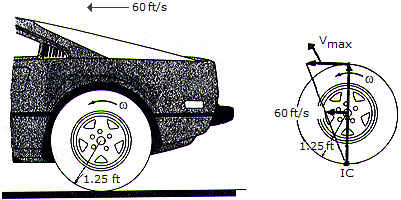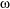# Engineering Mechanics - Planar Kinematics of a Rigid Body (PKRB) - Discussion

### Discussion :: Planar Kinematics of a Rigid Body (PKRB) - General Questions (Q.No.22)

22.The automobile with wheels 2.5 ft in diameter is traveling in a straight path at a rate of 60 ft/s. If no slipping occurs, determine the angular velocity of one of the rear wheels and the velocity of the fastest moving point on the wheel.

 [A].= 48 rad/s, vmax = 84.9 ft/s [B].= 24 rad/s, vmax = 84.9 ft/s [C].= 48 rad/s, vmax = 120.0 ft/s [D].= 24 rad/s, vmax = 120.0 ft/s

Explanation:

No answer description available for this question.

 Akim said: (Mar 19, 2017) I know that to find the vmax acceleration must be 0. Which equation should be used?